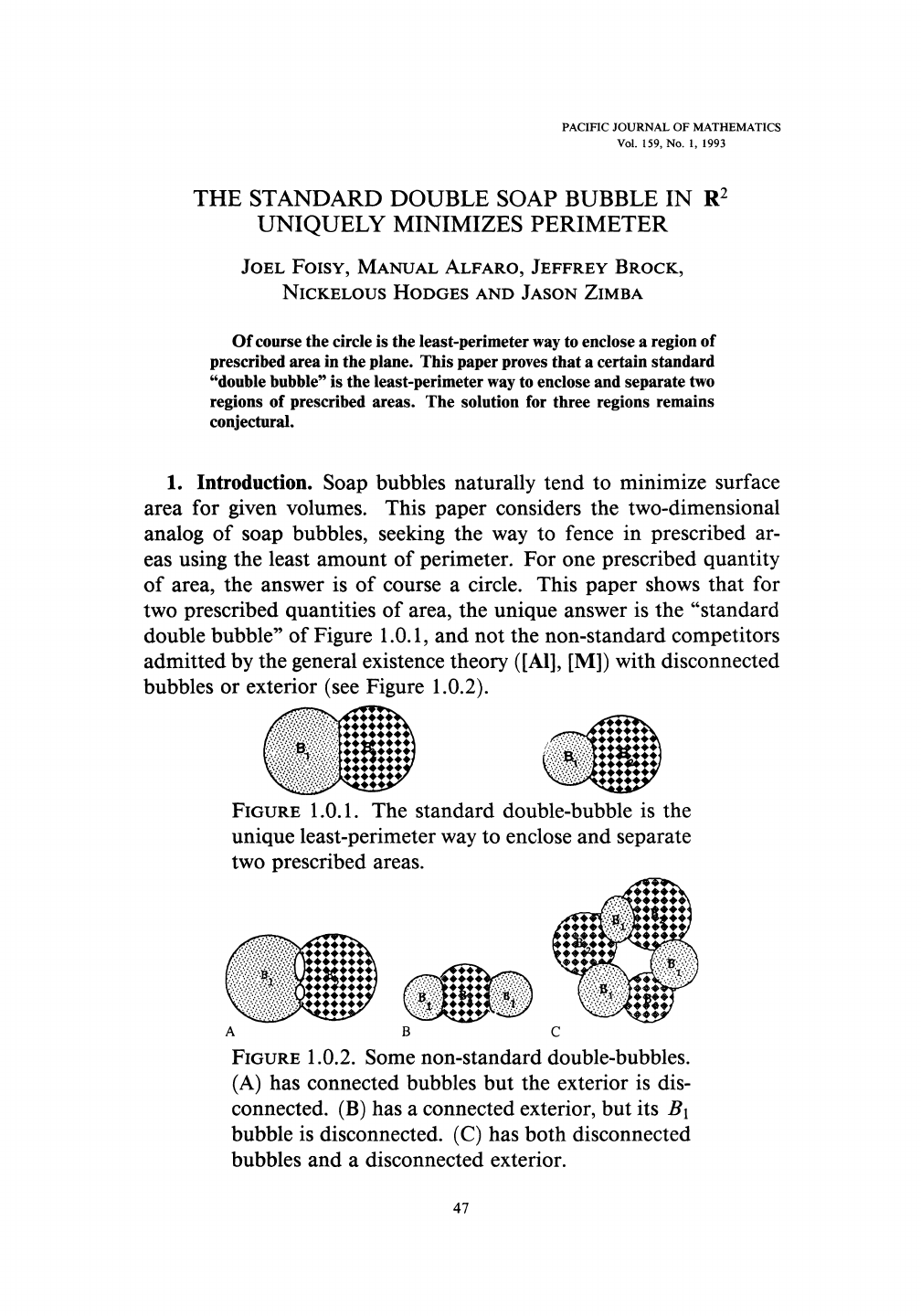A double bubble is pair of bubbles which intersect and are separate...
Here's an interesting way to prove the circle is the shape of maxim...
The proof for ℝ³ was actually achieved 2 years later by [Joel Hass,...
To calculate R, A and L let's first draw the entire circumference. ...
If the cluster is not connected as can be seen in the image on the ...
Here's a plot of A''(x) ![](https://i.imgur.com/VSAV95G.png) ...
Here's an illustration of the calculation of the total perimeter fo...PACIFIC JOURNAL OF MATHEMATICS
Vol. 159, No. 1, 1993
THE STANDARD DOUBLE SOAP BUBBLE IN R
2
UNIQUELY MINIMIZES PERIMETER
JOEL
FOISY,
MANUAL
ALFARO,
JEFFREY
BROCK,
NICKELOUS
HODGES
AND
JASON
ZIMBA
Of course the circle is the least-perimeter way to enclose a region of
prescribed area in the plane. This paper proves that a certain standard
"double bubble" i s the least-perimeter way to enclose and separate two
regions of prescribed areas. The solution for three regions remains
conjectural.
1.
Introduction. Soap bubbles naturally tend to minimize surface
area for given volumes. This paper considers the two-dimensional
analog of soap bubbles, seeking the way to fence in prescribed ar-
eas using the least amount of perimeter. For one prescribed quantity
of area, the answer is of course a circle. This paper shows that for
two prescribed quantities of area, the unique answer is the "standard
double bubble" of Figure 1.0.1, and not the non-standard competitors
admitted by the general existence theory
([Al],
[M]) with disconnected
bubbles or exterior (see Figure
1.0.2).
FIGURE
1.0.1. The standard double-bubble is the
unique least-perimeter way to enclose and separate
two prescribed areas.
A B C
FIGURE
1.0.2.
Some non-standard double-bubbles.
(A) has connected bubbles but the exterior is dis-
connected. (B) has a connected exterior, but its B\
bubble is disconnected. (C) has both disconnected
bubbles and a disconnected exterior.
47